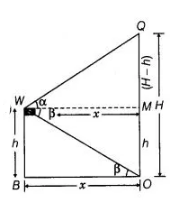# A window of a house is h m above the ground.

Question:

A window of a house is h m above the ground. Form the window, the angles of elevation and depression of the top and the bottom of another house

situated on the opposite side of the lane are found to be a and p, respectively. Prove that the height of the other house is h(1+tanα cot β)m.

Solution:

Let the height of the other house $=O Q=H$

and $\quad O B=M W=x \mathrm{~m}$

Given that, height of the first house $=W B=h=M O$

and $\angle Q W M=\alpha, \angle O W M=\beta=\angle W O B$

[alternate angle]Now, in $\Delta W O B, \quad \tan \beta=\frac{W B}{O B}=\frac{h}{x}$

$\Rightarrow$ $x=\frac{h}{\tan \beta}$ ...(i)

And in $\triangle Q W M$, $\tan \alpha=\frac{Q M}{W M}=\frac{O Q-M O}{W M}$

$\Rightarrow$ $\tan \alpha=\frac{H-h}{x}$

$\Rightarrow$ $x=\frac{H-h}{\tan \alpha}$ ... (ii)

From Eqs. (i) and (ii),

$\frac{h}{\tan \beta}=\frac{H-h}{\tan \alpha}$

$\Rightarrow \quad h \tan \alpha=(H-h) \tan \beta$

$\Rightarrow \quad h \tan \alpha=H \tan \beta-h \tan \beta$

$\Rightarrow \quad H \tan \beta=h(\tan \alpha+\tan \beta)$

$\therefore$ $H=h\left(\frac{\tan \alpha+\tan \beta}{\tan \beta}\right)$

$=h\left(1+\tan \alpha \cdot \frac{1}{\tan \beta}\right)=h(1+\tan \alpha \cdot \cot \beta)\left[\because \cot \theta=\frac{1}{\tan \theta}\right]$

Hence, the required height of the other house is $h(1+\tan \alpha \cdot \cot \beta)$

Hence proved.